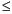Functions and CALL Routines

# PROBNEGB Function

Returns the probability from a negative binomial distribution.
 Category: Probability See: CDF Function

## Syntax

 PROBNEGB(p,n,m)

### Arguments

p

is a numeric probability of success parameter.

 Range: 0p1
n

is an integer number of successes parameter.

 Range: n1
m

is a positive integer random variable, the number of failures.

 Range: m0

The PROBNEGB function returns the probability that an observation from a negative binomial distribution, with probability of success p and number of successes n, is less than or equal to m.

To compute the probability that an observation is equal to a given value m, compute the difference of two probabilities from the negative binomial distribution for m and m-1.

SAS Statements Results
`x=probnegb(0.5,2,1);`
`0.5`

 Functions:Previous Page | Next Page | Top of Page